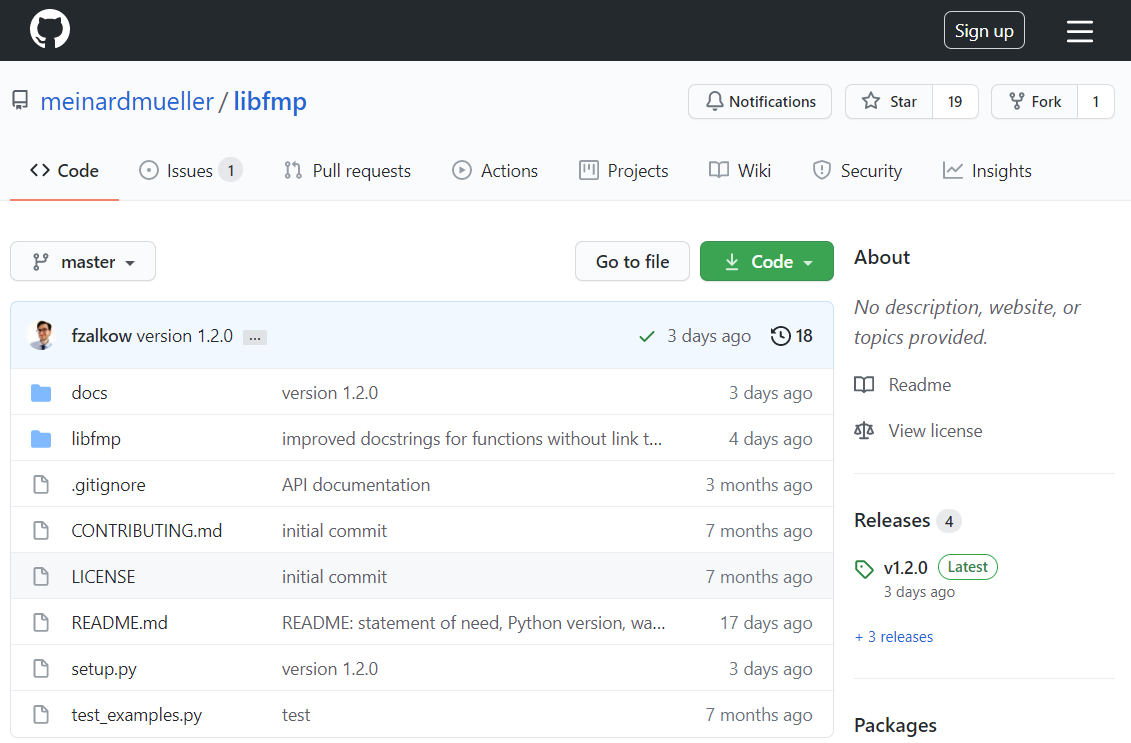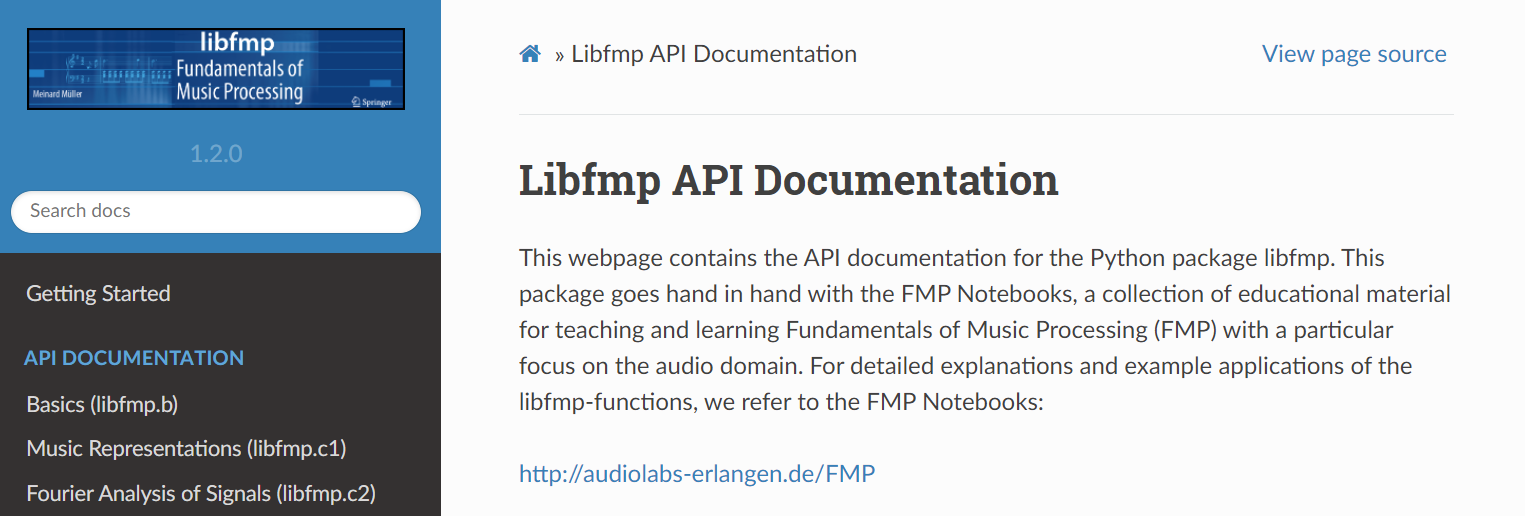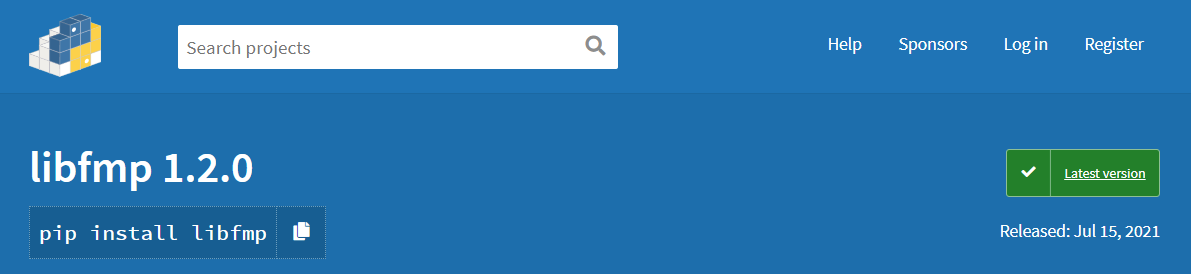This notebook gives a short introduction to the libfmp-package, the Python package that accompanies the FMP notebooks. After a short introduction to Python modules and packages, we explain how to the libfmp-package is structured and how it can be used. The libfmp-package is also provided at GitHub and PyPi (being installable via pip install libfmp). Further details can be found below and in the following publication:

• Meinard Müller and Frank Zalkow: libfmp: A Python Package for Fundamentals of Music Processing. The Journal of Open Source Software (JOSS), 6(63), 2021.

## Python Modules¶

A Python module is basically a file with an extension .py containing Python code. The content of a module can be accessed with the import statement. As an example, we consider the file b_test_module.py contained in the folder libfmp/B/. When the import statement is executed, the interpreter searches for b_test_module.py in a list of directories which specifies the search paths for modules. The variable sys.path (which is part of the module sys) yields the list of directories. It is initialized from the environment variable PYTHONPATH (plus an installation-dependent default). The list contained in sys.path can be extended using the function sys.path.append. The following example illustrates these concepts:

In :
import sys
print(sys.path, '\n')

import os
sys.path.append('..')
print(sys.path, '\n')

['/home/swpffm/dev/FMP_GroupMM/FMP/B', '/home/swpffm/miniconda3/envs/FMP/lib/python37.zip', '/home/swpffm/miniconda3/envs/FMP/lib/python3.7', '/home/swpffm/miniconda3/envs/FMP/lib/python3.7/lib-dynload', '', '/home/swpffm/miniconda3/envs/FMP/lib/python3.7/site-packages', '/home/swpffm/miniconda3/envs/FMP/lib/python3.7/site-packages/IPython/extensions', '/home/swpffm/.ipython']

['/home/swpffm/dev/FMP_GroupMM/FMP/B', '/home/swpffm/miniconda3/envs/FMP/lib/python37.zip', '/home/swpffm/miniconda3/envs/FMP/lib/python3.7', '/home/swpffm/miniconda3/envs/FMP/lib/python3.7/lib-dynload', '', '/home/swpffm/miniconda3/envs/FMP/lib/python3.7/site-packages', '/home/swpffm/miniconda3/envs/FMP/lib/python3.7/site-packages/IPython/extensions', '/home/swpffm/.ipython', '..']



Once the directory of the module is in the search path, we can use the import statement. Let us come back to our example test_module.py, which has the following content:

In :
import os

fn = os.path.join('..', 'libfmp', 'b', 'b_test_module.py')
with open(fn, 'r', encoding='utf-8') as stream:

print(content_text)

"""
Module: libfmp.b.b_test_module
Author: Meinard Mueller

This file is part of the FMP Notebooks (https://www.audiolabs-erlangen.de/FMP).
"""

string = 'This is a test function'
a, b, c = 1, 2, 3

def add(a, b=0, c=0):
"""Function to add three numbers

| Notebook: B/B_libfmp.ipynb and
| Notebook: B/B_PythonBasics.ipynb

Args:
a (float): First number
b (float): Second number (default: 0)
c (float): Third number (default: 0)

Returns:
d (float): Sum
"""
d = a + b + c
print('Addition: ', a, ' + ', b, ' + ', c, ' = ', d)
return d



The following options import the module b_test_module or some of its elements:

In :
import libfmp.b.b_test_module
libfmp.b.b_test_module.b,
libfmp.b.b_test_module.c)

from libfmp.b.b_test_module import add
result = add(4, 5)

from libfmp.b.b_test_module import add as s
result = s(6)

from libfmp.b.b_test_module import *
result = add(a, b, c)

Addition:  1  +  2  +  3  =  6
Addition:  4  +  5  +  0  =  9
Addition:  6  +  0  +  0  =  6
Addition:  1  +  2  +  3  =  6


The file variable test_module.__file__ determines the path where the module was found. Furthermore, when a .py-file is imported as a module, Python sets the variable __name__ to the name of the module. Finally, the help-function shows the documentation of the specified module.

In :
print('Directory of module:', libfmp.b.b_test_module.__file__)
print('Name of module:', libfmp.b.b_test_module.__name__)
print('=======================================')
help(libfmp.b.b_test_module)

Directory of module: ../libfmp/b/b_test_module.py
Name of module: libfmp.b.b_test_module
=======================================
Help on module libfmp.b.b_test_module in libfmp.b:

NAME
libfmp.b.b_test_module

DESCRIPTION
Module: libfmp.b.b_test_module
Author: Meinard Mueller

This file is part of the FMP Notebooks (https://www.audiolabs-erlangen.de/FMP).

FUNCTIONS
Function to add three numbers

| Notebook: B/B_libfmp.ipynb and
| Notebook: B/B_PythonBasics.ipynb

Args:
a (float): First number
b (float): Second number (default: 0)
c (float): Third number (default: 0)

Returns:
d (float): Sum

DATA
a = 1
b = 2
c = 3
string = 'This is a test function'

FILE
/home/swpffm/dev/FMP_GroupMM/FMP/libfmp/b/b_test_module.py



Note that any .py-file that contains a module can be also be executed as a Python script (e.g., python test_module.py). In the case that a file is run as a script, the variable __name__ is set to the string '__main__'. This allows for placing additional statements in the module that are executed only when being run as a script (and not when imported as a module). For example, one can place these elements in a conditional (if) block as follows:

if (__name__ == '__main__'):
Statements only executed when run as a script

## Python Packages¶

A Python package is a namespace that consists of a directory, which in turn may contain subdirectories (subpackages) and files (modules). The naming convention follows the hierarchical file structure using dot notation. Opposed to normal directories, a package in Python typically contains a particular file called __init__.py (until Python 3.3, the existence of such a file was even mandatory). This file is automatically executed when the package (or a module in the package) is imported. For example, this allows for initializing package-specific data or for automatically importing specific modules from a package.

Continuing our example above, the directory B can be regarded as a package containing an initialization file __init__.py. The following examples show how to import packages and modules:

In :
import sys
import os
sys.path.append('..')

import libfmp.b.b_test_module
libfmp.b.b_test_module.b,
libfmp.b.b_test_module.c)

from libfmp.b import b_test_module
result = b_test_module.add(b_test_module.a, b_test_module.b, b_test_module.c)

Addition:  1  +  2  +  3  =  6
Addition:  1  +  2  +  3  =  6


## The Package libfmp¶

In the FMP notebooks, the naming conventions and the code structure are carefully matched to the mathematical notation used in the textbook [Müller, FMP, Springer 2015] to establish a close relationship between theory and practice. Furthermore, the programming style is kept explicit and straightforward with a flat, functional hierarchy. Most code examples are directly specified in the code cells interleaved with text cells containing explanations. This approach leads to redundancies, where individual code fragments may occur several times in the FMP notebooks. In this sense, the FMP notebooks are not designed to serve as a toolbox per se. Nevertheless, we have assembled a Python package called libfmp, which serves several purposes.

• First, libfmp contains some simple reference implementation for the most essential functionalities introduced in the FMP notebooks.
• Second, the package allows for reducing some redundancies in the FMP notebook at places where implementation issues are not the focus.
• Third, we hope that libfmp provides some instructive examples on how to document and build up modules, packages, and libraries in Python.

The package libfmp is organized along with the structure of the FMP notebooks. Containing subpackages called B, C1, C2, ..., C8, the file structure of libfmp is as follows:

libfmp
├── __init__.py
├── C1
│   ├── __init__.py
│   ├── ...
⋮
└── C8
├── __init__.py
├── ...

The package libfmp is located in the main directory of the FMP notebooks (which is the parent directory of the current notebook B_libfmp.ipynb). Therefore, to access libfmp from the current path, we need to add the parent directory ('..') to Python's search path. In the following example, the package libfmp is imported and its help page is displayed.

In :
import sys
sys.path.append('..')
import libfmp
help(libfmp)

Help on package libfmp:

NAME
libfmp

PACKAGE CONTENTS
b (package)
c1 (package)
c2 (package)
c3 (package)
c4 (package)
c5 (package)
c6 (package)
c7 (package)
c8 (package)

FILE
/home/swpffm/dev/FMP_GroupMM/FMP/libfmp/__init__.py



The __init__.py-file of libfmp is empty. This means that no functionality is available at this stage. To import the modules contained in the subpackages, one needs to import the subpackages individually. The __init__.py files of the subpackages B, C1, C2, ..., C8 are not empty; they contain a list of all functions that are considered essential for a user of the FMP notebooks and libfmp. In the following example, the subpackage C8 is imported, and the help page is displayed.

In :
import sys
sys.path.append('..')
from libfmp import c8
help(c8)

Help on package libfmp.c8 in libfmp:

NAME
libfmp.c8

PACKAGE CONTENTS
c8s1_hps
c8s2_f0
c8s2_salience
c8s3_nmf

FILE
/home/swpffm/dev/FMP_GroupMM/FMP/libfmp/c8/__init__.py



Next, we show the content of __init__.py of C8.

In :
import os
fn = os.path.join('..', 'libfmp', 'c8', '__init__.py')
with open(fn, 'r', encoding='utf-8') as stream:
print(content_text)

from .c8s1_hps import median_filter_horizontal, \
median_filter_vertical, \
convert_l_sec_to_frames, \
convert_l_hertz_to_bins, \
make_integer_odd, \
hps, \
generate_audio_tag_html_list, \
hrps, \
experiment_hrps_parameter

from .c8s2_salience import principal_argument, \
compute_if, \
f_coef, \
frequency_to_bin_index, \
p_bin, \
compute_y_lf_bin, \
p_bin_if, \
compute_salience_rep

from .c8s2_f0 import hz_to_cents, \
cents_to_hz, \
sonify_trajectory_with_sinusoid, \
visualize_salience_traj_constraints, \
define_transition_matrix, \
compute_trajectory_dp, \
convert_ann_to_constraint_region, \
compute_trajectory_cr, \
compute_traj_from_audio, \
separate_melody_accompaniment

from .c8s3_nmf import nmf, \
plot_nmf_factors, \
pitch_from_annotation, \
template_pitch, \
init_nmf_template_pitch, \
init_nmf_activation_score, \
init_nmf_template_pitch_onset, \
init_nmf_activation_score_onset



Note that using __init__.py allows a user to access the specified functions without the need to specify the filenames the functions are contained in. For example, the function c8.c8s1_hpa.hps can be directly accessed via c8.hps. Besides its easier access, the convention also allows users to easily rename the filename c8s1_hps with a single update in the __init__.py without any further changes in the code.

## Documentation of Functions¶

For documenting the functions contained in libfmp, we follow standard Python style conventions as formulated in the Google Python Style Guide. Most of the libfmp-functions are contained in some FMP notebook, where one finds a detailed explanation of the application, the underlying theory, and implementation issues. The FMP notebooks also provide illustrative examples, experiments with different parameter settings, and a discussion of results. In the Docstring of a libfmp-function, we specify the FMP notebook where the function is explained and developed. Using the help-function, the following example shows the docstring of the function libfmp.c8.hps. In particular, the information Notebook: C8/C8S1_HPS.ipynb shows that this function is introduced in the FMP notebook on harmonic–percussive separation (HPS) with the filename C8/C8S1_HPS.ipynb.

In :
help(c8.hps)

Help on function hps in module libfmp.c8.c8s1_hps:

hps(x, Fs, N, H, L_h, L_p, L_unit='physical', mask='binary', eps=0.001, detail=False)
Harmonic-percussive separation (HPS) algorithm

Notebook: C8/C8S1_HPS.ipynb

Args:
x (np.ndarray): Input signal
Fs (scalar): Sampling rate of x
N (int): Frame length
H (int): Hopsize
L_h (float): Horizontal median filter length given in seconds or frames
L_p (float): Percussive median filter length given in Hertz or bins
L_unit (str): Adjusts unit, either 'pyhsical' or 'indices' (Default value = 'physical')
mask (str): Either 'binary' or 'soft' (Default value = 'binary')
eps (float): Parameter used in soft maskig (Default value = 0.001)
detail (bool): Returns detailed information (Default value = False)

Returns:
x_h (np.ndarray): Harmonic signal
x_p (np.ndarray): Percussive signal
details (dict): Dictionary containing detailed information; returned if detail=True



# The Package libfmp at GitHub¶

The Python package libfmp is also provided at GitHub, which is a code hosting platform for the free and open source distributed version control system Git. The packages libfmp is exported from the FMP notebooks in a fully automatic process and then pushed to the following GitHub repository:The folder libfmp in the GitHub repository coincides with the sources contained in the FMP notebooks' folder FMP/libfmp. The remaining files of the Github repository are also contained in the FMP notebooks' main folder (provided with the prefix libfmp_ which is removed in the automated export). The content of the folder docs contains sources for the libfmp API documentation, which is based on the documentation generation package sphinx. An HTML export of this documentation, is available at:## The Package libfmp at PyPi¶

The Python Package Index (PyPI) is a repository of software for the Python programming language, which helps users to find and install software developed and shared by the Python community. The package libfmp has been exported automatically to PyPi and is accessible at:The command pip is used to look for packages in PyPI, to calculate the packages' dependencies, and to install them. The PyPi-version of libfmp can be installed (within a given Python environment) in the following way:

pip install libfmp
Acknowledgment: This notebook was created by Meinard Müller and Frank Zalkow.